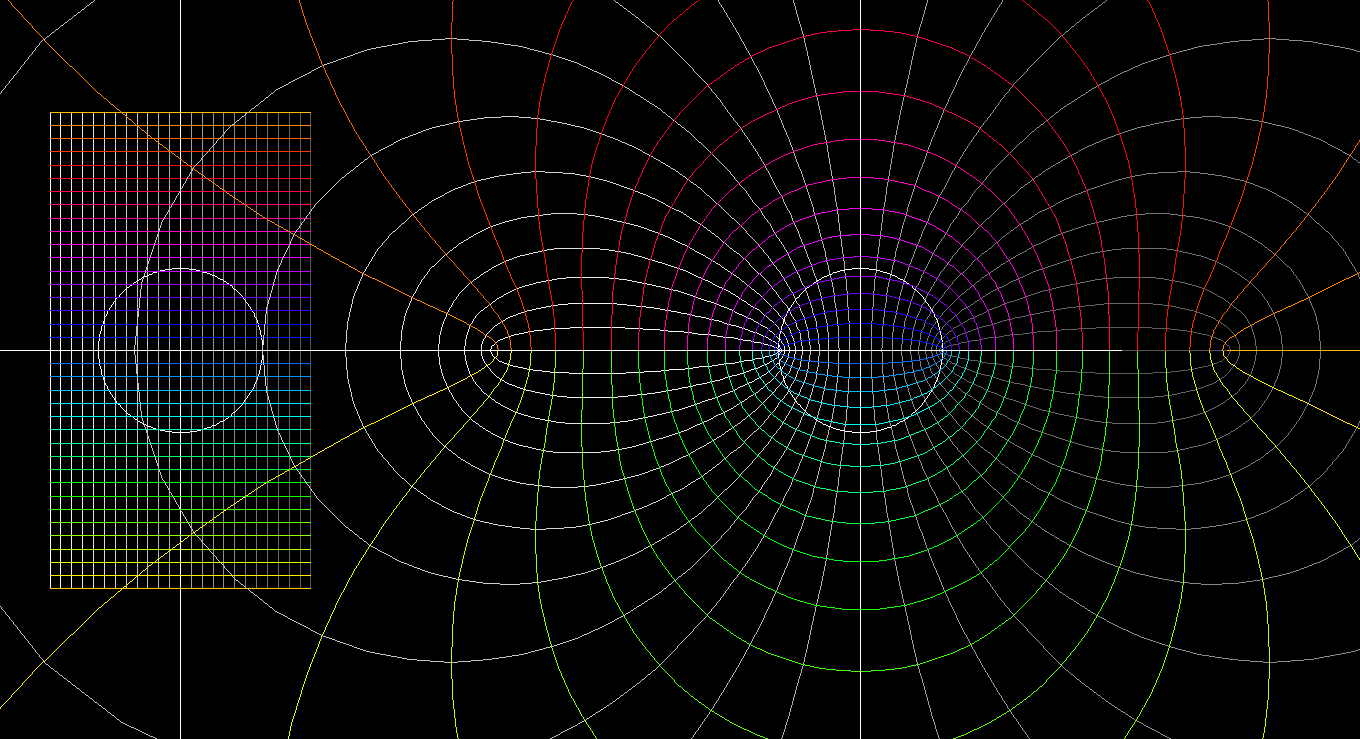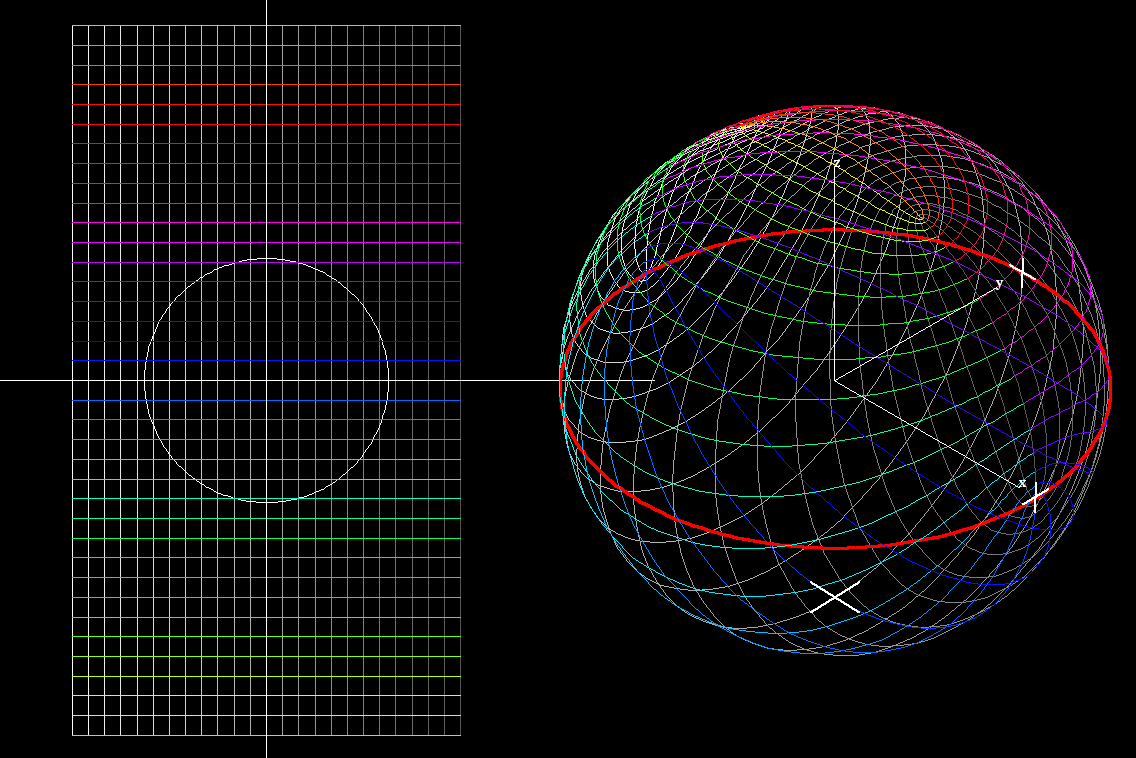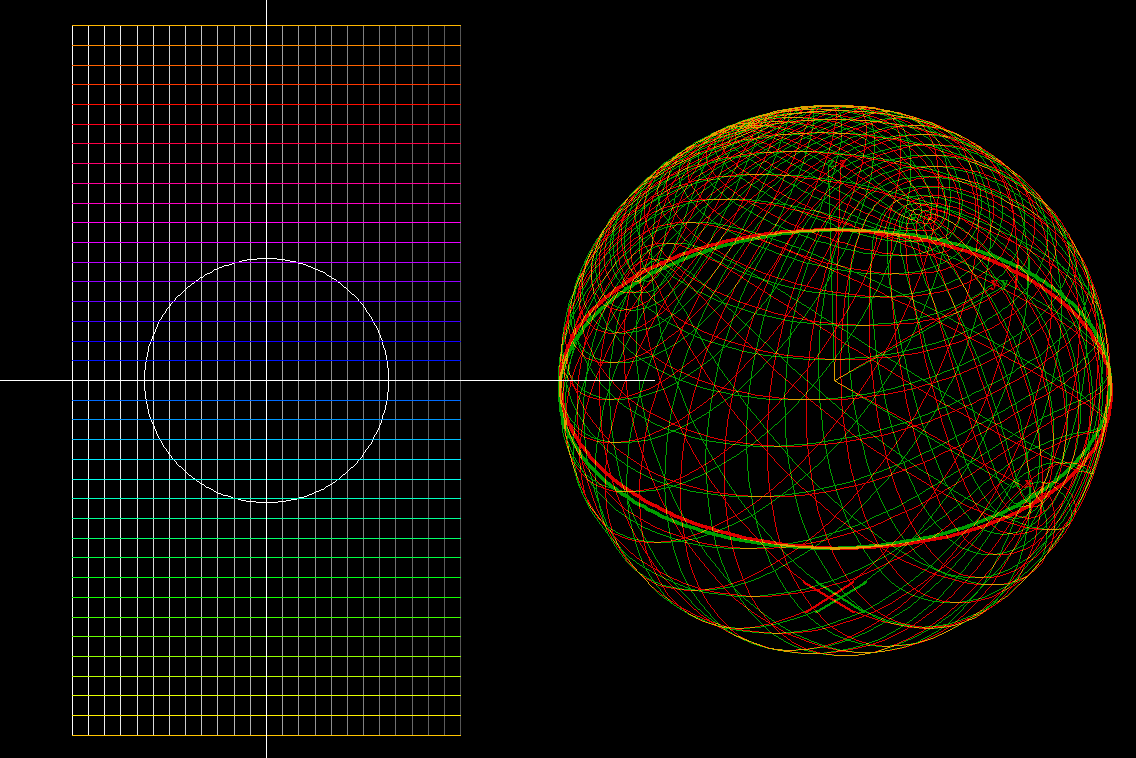# Jacobi sn Functionfunction: z → sn(z,m). This is a family of functions, one for each m. Each of the sn(.,m) is doubly periodic from C to C. We show one half of the fundamental domain for the period translations. This domain is mapped by sn(.,m) in a one-to-one way to C including infinity. Each function has a symmetry so that sn(.,m) has the same values again on the other half of the fundamental domain. The two infinite values are simple poles. In the animation we show domain and range for 0.05 ≦ m ≦ 0.7.function: z → sn(z,m) again, but here we show the values on the Riemann sphere. morph: 0.05 ≦ m ≦ 0.7. The symmetries of these functions are better seen on the Riemann sphere. One can clearly see the four branch values, that is, values sn(z,m) with sn'(z,m) = 0. For all m, two branch values are +1, -1, the other two move, but remain symmetric to the imaginary axis.function: z → sn(z,m) again, with values on the Riemann sphere. morph: 0.05 ≦ m ≦ 0.7. With red-green glasses for stereo viewing, the range image looks much clearer.